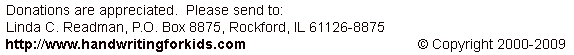Web www.handwritingforkids.com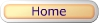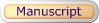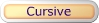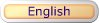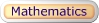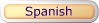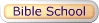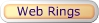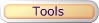### Mathematics - Multiplication

• Online / Interactive
• Printable w/guideline
• Printable
• Customized

This online/interactive math multiplication generator will provides plenty of online math practice, boost test scores and prepare the child/children to meet the Grades 3-5 curriculum standards. This online/interactive math multiplication, will create 1 digit by 1 digit (1x1) equations with an option of timed test, and it will automatically graded/scored your results in a printable worksheet.

Type of online math problems:

• Online Math 1 - Factor of Three (3)
• Online Math 1 - Factor of Six (6)
• Online Math 1 - Factors Zero to Four
• Online Math 1 - Factors Zero to Eight
• and many more!
 3 x 3 9This printable basic math multiplication by factors worksheet generator will provides plenty of math practice, boost test scores and prepare the child/children to meet the Grades 3-5 curriculum standards. This math worksheet maker will create a vertical math multiplication, 1 digit by 1 digit (1x1) equations with handwriting lines.

See sample math worksheets below:

 3 x  3 9

This printable basic math multiplication by factors worksheet generator will provides plenty of math practice, boost test scores and prepare the child/children to meet the Grades 3-5 curriculum standards. This math worksheet maker will create a vertical math multiplication, 1 digit by 1 digit (1x1) equations.

See sample math worksheets below:

 2 x 3 6

This worksheet generator will create your own customized/personalized multiplication worksheet. You enter your own math problems in a printable worksheet. Maximum 5 digits x 5 digits (5x5).

 2 x   4 8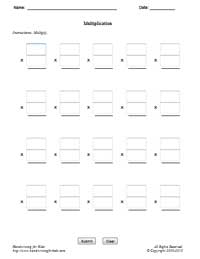• Online / Interactive
• Printable w/guideline
• Printable
• Customized

This online/interactive horizontal math multiplication, will create 1 digit by 1 digit (1x1) equations with an option of timed test, and it will automatically graded/scored your results in a printable worksheet.

Type of online math problems:

• Online Math 1 - Factor of Three (3)
• Online Math 1 - Factor of Six (6)
• Online Math 1 - Factors Zero to Four
• Online Math 1 - Factors Zero to Eight
• and many more!
 4 x 1 = 4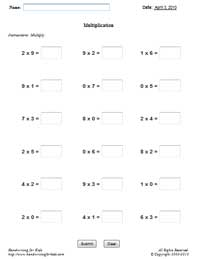This printable basic math multiplication by factors worksheet generator will provides plenty of math practice, boost test scores and prepare the child/children to meet the Grades 3-5 curriculum standards. This math worksheet maker will create a horizontal math multiplication, 1 digit by 1 digit (1x1) equations with handwriting lines.

See sample math worksheets below:

 1 x 5 = 5

This printable basic math multiplication by factors worksheet generator will provides plenty of math practice, boost test scores and prepare the child/children to meet the Grades 3-5 curriculum standards. This math worksheet maker will create a horizontal math multiplication, 1 digit by 1 digit (1x1) equations without handwriting lines.

See sample math worksheets below:

 6 x 3 = 18

This worksheet generator will create your own customized/personalized multiplication worksheet. You enter your own vertical math problems in a printable worksheet. Maximum 5 digits x 5 digits (5x5).

 3 x 4 = 12• Online / Interactive
• Printable w/guideline
• Printable
• Customized

This online/interactive math multiplication, will create 2 digits by 1 digit (2x1) and/or 1 digit by 2 digits (1x2) equations with an option of timed test, and it will automatically graded/scored your results in a printable worksheet. Suggestive Grade Levels: Grades 3-5

 17 x  5 85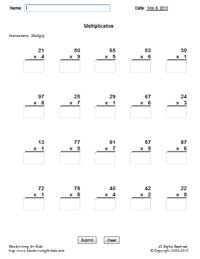This printable math multiplication worksheet generator will create a vertical math multiplication, 2 digits by 1 digit (2x1) and/or 1 digit by 2 digits (1x2) equations with handwriting lines.

 17 x  5 85

This printable math multiplication worksheet generator will create a vertical math multiplication, 2 digits by 1 digit (2x1) and/or 1 digit by 2 digits (1x2) equations without the handwriting lines.

 17 x  5 85

This worksheet generator will create your own customized/personalized multiplication worksheet. You enter your own math problems in a printable worksheet. Maximum 5 digits x 5 digits (5x5).

 17 x  5 85### Multiplication - TablesExamples Multiplication Tables How to use the multiplication table Blank Table #1 Answer Sheet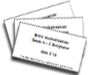Multiplication 0-12 Flash Cards (MS Word Document - Printable Only) Flash Cards Printing Instructions: 1. Print all odd pages 2. Flip the stack of printed pages and re-insert into the printer. 3. Print all even pages. Suggestion: Print on color paper or card-stock paper.

### JavaScript Interactive Math* - Multiplication

 JavaScript Math (Interactive)* Times Table - Worksheets Times Table - Answer Sheets

* JavaScript must be enabled on your browser.

Sudoku for Kids (Picture Sudoku) 4x4